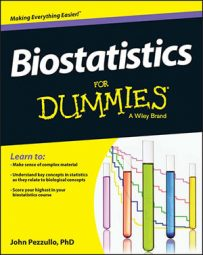##### Biostatistics For DummiesThe so-called “one-way analysis of variance” (ANOVA) is used when comparing three or more groups of numbers. When comparing only two groups (A and B), you test the difference (A – B) between the two groups with a Student t test. So when comparing three groups (A, B, and C) it’s natural to think of testing each of the three possible two-group comparisons (A – B, A – C, and B – C) with a t test.

But running an exhaustive set of two-group t tests can be risky, because as the number of groups goes up, the number of two-group comparisons goes up even more. The general rule is that N groups can be paired up in N(N – 1)/2 different ways, so in a study with six groups, you’d have 6×5/2, or 15 different two-group comparisons.

When you do a lot of significance tests, you run an increased chance of making a Type I error — falsely concluding significance when there’s no real effect present. This type of error is also called an alpha inflation. So if you want to know whether a bunch of groups all have consistent means or whether one or more of them are different from one or more others, you need a single test producing a single p value that answers that question.

The one-way ANOVA is exactly that kind of test. It doesn’t look at the differences between pairs of group means; instead, it looks at how the entire collection of group means is spread out and compares that to how much you might expect those means to spread out if all the groups were sampled from the same population (that is, if there were no true differences between the groups).

The result of this calculation is expressed in a test statistic called the F ratio (designated simply as F), the ratio of how much variability there is between the groups relative to how much there is within the groups.

If the null hypothesis is true (in other words, if no true difference exists between the groups), then the F ratio should be close to 1, and its sampling fluctuations should follow the Fisher F distribution, which is actually a family of distribution functions characterized by two numbers:

• The numerator degrees of freedom: This number is often designated as dfN or df1, which is one less than the number of groups.

• The denominator degrees of freedom: This number is designated as dfD or df2, which is the total number of observations minus the number of groups.

The p value can be calculated from the values of F, df1, and df2, and the software will perform this calculation for you. If the p value from the ANOVA is significant (less than 0.05 or your chosen alpha level), then you can conclude that the groups are not all the same (because the means varied from each other by too large an amount).